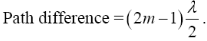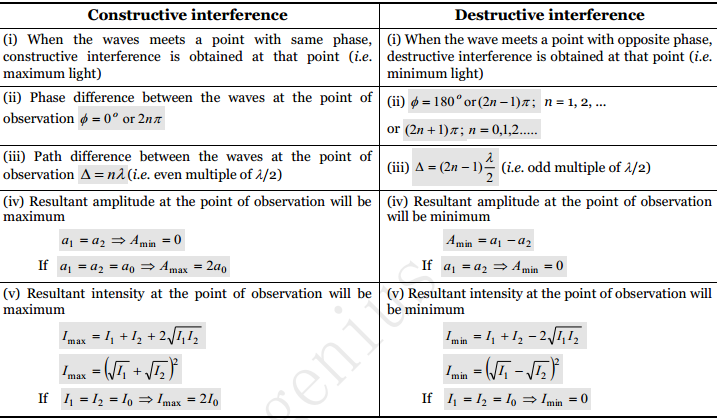### Interference of Light Waves

When two light waves of same frequency travel simultaneously in the same direction then, due to their superposition, the resultant intensity of light at any point in space is different from the sum of intensities of two waves. At some points the resultant intensity is maximum while at some points it is minimum. The re-distribution of light intensity due to the superposition of two light waves is called interference of light.

The interference is said to constructive where the resultant intensity is constructive and destructive at points where the resultant intensity is minimum or zero.

### Conditions for Interference

Let the source be the monochromatic and S1 and S2 be the two similar parallel sits very close together from source. The two sources are in same phase.

Let a1 and a2 be the amplitudes of light waves from S1 and S2 respectively.

The waves arrive at P having traversed different paths S1P and S2P. Hence they are superposed at P with a phase difference ϕ, given by

Where x is the path difference (S1P and S2P) between the waves at P and λ is the wavelength of light. The displacement at P due to the simple harmonic waves from S1 and S2 may then e represented by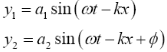And ω/2π is the frequency of each wave.

By the principle of superposition, the resultant displacement a position at P is given by,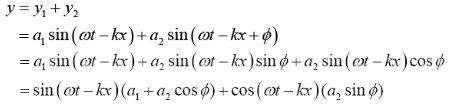On further solving,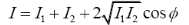Thus, the resultant intensity at any point depends upon the phase difference ϕ between the two waves at that point.

### For constructive interference: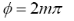Thus, at points where the two interfering waves meet in the same phase (that is, the phase difference between them is 0, 2π, 4π….) the resultant intensity is maximum. This is constructive interference.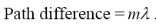### For destructive interference:

Thus, at points where the two interfering waves meet in the opposite phase (that is, the phase difference between them is 0, 3π, 5π….) the resultant intensity is minimum.